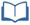# Teacher Resources

## A Deeper Look at Exponential Functions

On this page you will find lesson objectives, standards, instructional notes and UDL opportunities.

Estimated Time:  30 - 40 minutes

###Lesson Objectives:

• Students will apply understanding of residuals and functional behavior to determine whether an exponential function model is the best fit for a data set.
• Students find an appropriate exponential model to fit a data set, including models that require vertical translations.
• Students will identify if the context of the situation requires discrete or continuous data.

Key Common Core State Standards:

S.ID.6 Represent data on two quantitative variables on a scatter plot and describe how the variables are related.

1. Fit a function to the data; use functions fitted to data to solve problems in the context of the data.

Supporting Common Core State Standards:
F.IF.4 For a function that models a relationship between two quantities, interpret key features of graphs and tables in terms of the quantities, and sketch graphs showing key features given a verbal description of the relationship.

F.IF.7 Graph functions expressed symbolically and show key features of the graph, by hand in simple cases and using technology for more complicated cases.

Standards for Mathematical Practice Emphasized:

MP.1 Make sense of problems and persevere in solving them.
MP.2 Reason abstractly and quantitatively.
MP.3 Construct viable arguments and critique the reasoning of others.
MP.4 Model with mathematics.
MP.5 Use appropriate tools strategically.
MP.6 Attend to precision.

## Instructional Notes:

In this lesson, students will expand their understanding of exponential functions to cases that require vertical translations of a regression equation.  Students will evaluate exponential models to determine if all values make sense in the context of the scenario, thus distinguishing between discrete and continuous exponential models.

> Go to A Deeper Look at Exponential Functions lesson

## Sample Responses

For sample responses to the Algebra II Journal questions, visit the Algebra II Journal in Teacher Resources.

## A Deeper Look at Exponential Functions - Page 6

The second question requires students to find the x-value when y = 75.  If students have not studied logarithms in depth, they can use graphing to solve.  If they are studying exponential and logarithmic functions, this would be a time to encourage students to solve using logarithms.

> Go to lesson, page 6

## A Deeper Look at Exponential Functions - Page 11

As an optional extension or in-class activity, have students collect cooling curve data for other heated beverages and compare.  Use this opportunity to practice calculating an exponential regression equation and calculating residuals to analyze the fit of the model.

> Go to lesson, page 11

## A Deeper Look at Exponential Functions - Page 12

For sample responses to the Algebra II Journal questions, visit the Algebra II Journal in Teacher Resources.

> Go to lesson, page 12

## UDL Opportunities:

Checkpoint 3.1: Activate or supply background knowledge.
Checkpoint 3.2: Highlight patterns, critical features, big ideas, and relationships.
This lesson allows students to build on their prior understanding of exponential functions to include special cases. Students need to activate background knowledge to verify that an exponential model is appropriate for the contexts provided.

Checkpoint 3.3: Guide information processing, visualization, and manipulation.
Checkpoint 3.4: Maximize transfer and generalization.
Students are given an exponential model for which a standard exponential regression does not fit the data.  Upon inspection students should still conclude that an exponential model is appropriate, so a new strategy will be necessary to find the model of best fit.  The lesson demonstrates how to account for a vertical translation of an exponential graph.

Checkpoint 4.1: Vary the methods for response and navigation.
Checkpoint 7.1: Optimize individual choice and autonomy.
Checkpoint 7.2: Optimize relevance, value, and authenticity.

The scenarios in this lesson are based on real-world data in order to optimize relevance and authenticity.  The optional in-class extension offers students an opportunity to collect and analyze real-world data.